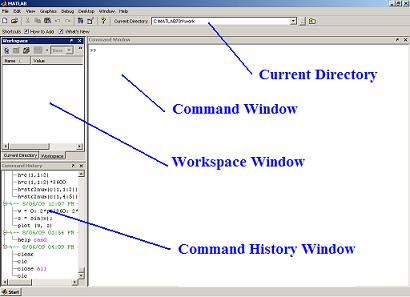Tutorial Lesson: Using MATLAB (a minimum session)

 To start using Matlab, the most important things to learn are how to enter matrices, how to use the ':' (colon) operator, and how to invoke functions. After you understand the basics (in the Tutorials Section), you can access the rest of the site as needed, or you can use the online help and demonstrations to learn the (many) other commands.

You'll log on or invoke MATLAB, do a few trivial calculations and log off.

Launch MATLAB. You can start it from your Start Menu, as any other Windows-based program. You see now something similar to this Figure:The History Window is where you see all the already typed commands. You can scroll through this list.

The Workspace Window is where you can see the current variables.

The Comand Window is your main action window. You can type your commands here.

At the top of the window, you can see your current directory. You can change it to start a new project.

Once the command window is on screen, you are ready to carry out this first lesson. Some commands and their output are shown below.

Enter 5+2 and hit the enter key. Note that the result of an unassigned expression is saved in the default variable 'ans'.

>> 5+2

ans =

7

The '>>' sign means that MATLAB is ready and waiting for your input.

You can also assign the value of an expression to a variable.

>> z=4

z =

4

A semicolon (at the end of your command) suppresses screen output for that instruction. MATLAB remembers your variables, though. You can recall the value of x by simply typing x

>> x=4;
>> x

x =

4

MATLAB knows trigonometry. Here is the cosine of 6 (default angles are in radians).

>> a=cos(6)

a =

0.9602

The floating point output display is controlled by the 'format' command. Here are two examples.

>> format long
>> a

a =

0.96017028665037

>> format short
>> a

a =

0.9602

Well done!
Close MATLAB (log off).
You can also quit by selecting 'Exit MATLAB' from the file menu.

From 'Using Matlab' to home

From 'Using Matlab' to 'Tutorials'

 Top Next: Working with vectors Video: writing Matlab Programs